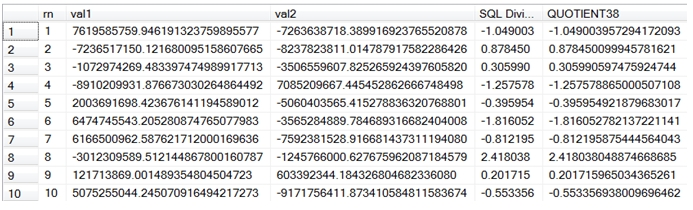# SQL Server QUOTIENT function with precision 38 and scale 18

QUOTIENT38

Updated: 30 November 2012

Use the scalar function QUOTIENT38 to return the quotient of 2 decimal(38,18) values as a decimal with precision 38 and scale 18. Numeric is functionally equivalent to decimal.
Syntax
SELECT [wctMath].[wct].[QUOTIENT38](
<@Val1, numeric(38,18),>
,<@Val2, numeric(38,18),>)
Arguments
@Val1
is the numerator in the division. @Val1 is an expression of type decimal(38,18) or of a type that can be implicitly converted to decimal(38,18)
@Val2
is the denominator in the division. @Val2 is an expression of type decimal(38,18) or of a type that can be implicitly converted to decimal(38,18)
Return Types
[numeric](38, 18)
Remarks
·         The maximum value returned by the function is 9999999999999999999.999999999999999999.
·         The minimum value returned by the function is -9999999999999999999.999999999999999999
·         If the quotient of @Val1 / @Val2 is greater than the maximum value or less than the minimum value, a NULL will be returned.
·         For multiplication, use the PRODUCT38 function.
Example
This example demonstrates the difference in results between standard decimal multiplication in SQL Server and the QUOTIENT38 function.
CREATE TABLE #q(
rn          int,
val1 decimal(38,18),
val2 decimal(38,18)
)

INSERT INTO #q
SELECT *
FROM (VALUES
(1,7619585759.946191323759895577,-7263638718.389916923765520878),
(2,-7236517150.121680095158607665,-8237823811.014787917582286426),
(3,-1072974269.483397474989917713,-3506559607.825265924397605820),
(4,-8910209931.876673030264864492,7085209667.445452862666748498),
(5,2003691698.423676141194589012,-5060403565.415278836320768801),
(6,6474745543.205280874765077983,-3565284889.784689316682404008),
(7,6166500962.587621712000169636,-7592381528.916681437311194080),
(8,-3012309589.512144867800160787,-1245766000.627675962087184579),
(9,121713869.001489354804504723,603392344.184326804682336080),
(10,5075255044.245070916494217273,-9171756411.873410584811583674)
) n(rn,val1, val2)

SELECT *
,val1 / val2 as [SQL Divide]
,wct.QUOTIENT38(Val1, val2) as QUOTIENT38
FROM #q

DROP TABLE #q
This produces the following result.### SupportCopyright 2008-2021 Westclintech LLC         Privacy Policy        Terms of Service